# Pandas Transform and Filter

In this blog we will see how to use Transform and filter on a groupby object. We all know about aggregate and apply and their usage in pandas dataframe but here we are trying to do a Split - Apply - Combine. We want to split our data into groups based on some criteria, then we apply our logic to each group and finally we combine the data back together into a single data frame. What does it mean?

### OriginalData

This is the Original table on which we will see how to perform Split - Apply - Combine using Transform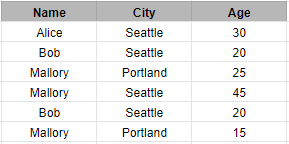### Split

Split the data by grouping on Name and City. Here we have formed 4 groups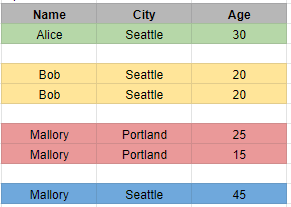### Apply

For each of these groups we want to add the ages and find the combined sum for each group. For example: group Bob and Seattle has a sum of 40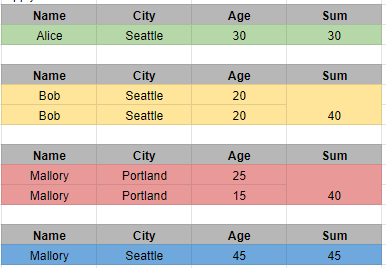### Combine

After combined sum of each group is calculated then we add a new column called Sum in the original dataframe, which will represent each row and their corresponding sum of Ages in the Group. For example: Bob, Seattle has two rows and each row has value of Sum column as 40, which represents their sum of Ages in the Group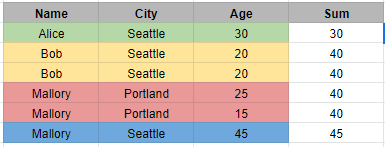Let’s see how we can achieve this using Pandas. We would be using the Transform function to create a new column Sum.

## Create Dataframe

``````import pandas as pd
import numpy as np
df = pd.DataFrame( {
"Name" : ["Alice", "Bob", "Mallory", "Mallory", "Bob" , "Mallory"] ,
"City" : ["Seattle", "Seattle", "Portland", "Seattle", "Seattle",
"Portland"] ,
"Age" : [30, 20, 25, 45, 20 , 15]} )
df
``````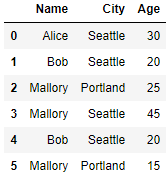## Use Apply

Lets see what are the different methods using which we can achieve this in Pandas. First we are going to apply sum on this dataframe and see what is the result. Apply returns a Series of size 4, but the original dfs length is 6. if you want to answer What is the sum of ages for each Individual and City, then the apply method is the more suitable one to choose. Here it gives the Name, City and their combined(sum) Ages

``````df.groupby(['Name','City'])['Age'].apply(sum).reset_index()
or
df.groupby(['Name','City'])['Age'].sum().reset_index()
``````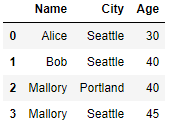## Pandas Groupby Transform

Let’s use Transform to add this combined(sum) Ages in each group to the original dataframe rows. This is what exactly the result that we were looking for. All the rows with same Name and City are grouped first and then sum up the Ages in each group and then enter this total sum in the column Sum

``````df['sum']=df.groupby(['Name','City'])['Age'].transform('sum')
df
``````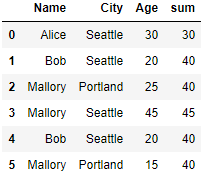## How to use Transform to filter the data?

Transform can also be used to filter the data. Here we are trying to get rows where column Sum value is greater than 40

``````df[df.groupby(['Name','City'])['Age'].transform('sum') > 40]
``````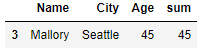## How to use Transform with Lambda?

We can also use transform with Lambda or any custom function. which is use for transforming the data. If a function, must either work when passed a DataFrame or when passed to DataFrame.apply . Here we are trying to divide the combined Ages by 2 (half of the group combined age). Look at Bob, Seattle row , we have seen their combined age in the group was 40 and after applying lambda with transform the new column half_age has half the value of Sum column i.e. 20

``````df['half_age']=df.groupby(['Name','City'])['Age'].transform(lambda x: x.sum()/2)
df
``````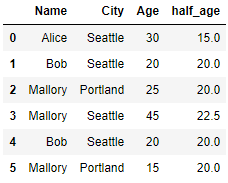## How to use Filter with Pandas Groupby ?

The filter method returns a subset of the original object. Suppose we want to take only elements that belong to groups with a group sum greater than 30. Group Bob, Seattle has group sum of 40 whereas Alice, Seattle group sum is 30 and that’s the reason it is not displayed in the result

``````df.groupby(['Name','City']).filter(lambda x: sum(x['Age']) > 30)
``````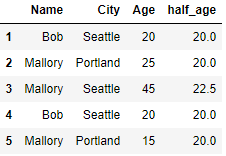Another useful operation is filtering out elements that belong to groups with only a couple members. So if I want only the groups which is having more than 1 row in it

``````df.groupby(['Name','City']).filter(lambda x: len(x) > 1)
``````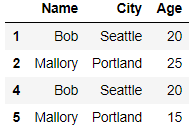Lets look at another option map to create the column sum in our original Dataframe.

## How to use Map to create a new Column?

``````df['Sum']=df.Name.map(df.groupby(['Name'])['Age'].sum())
df
``````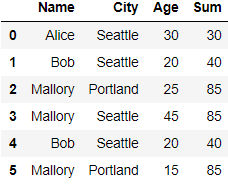I am just grouping on the Name column here and used map function to map the group sum of the Age

## Conclusion

In this post we have seen how we can use Transform to Split - Apply and Combine the results and additionally transform can be used to filter the records and can also be used in conjunction with Lambda and other custom Functions. The Filter function is used to get the records matching our criteria. And finally the map function which can also be used as an alternative to transform in order to create the new column with the group operations like sum, count, mean etc.

Tags:

Categories:

Updated: